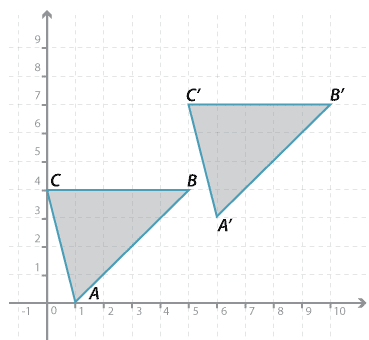#### Question 2

Use a column of two numbers to represent the vector of the translation shown below.Detailed description

 $$\Bigg[$$ value of x coordinate $$\Bigg]$$ value of y coordinate

#### Question 3

A triangle with vertices $$A(1, 7), B(4, 8), C(3, 2)$$ is translated '4 units to the right and 3 down'. Find the coordinates of the vertices of the image $$\triangle A^\prime B^\prime C^\prime$$.

Give the $$x$$-coordinate and the $$y$$-coordinate of:

$$x$$ = $$y$$ = Submit answer
a $$A^\prime$$
b $$B^\prime$$
c $$C^\prime$$

Place answers in both checkboxes before submitting.